# Math in Focus Grade 7 Chapter 3 Lesson 3.4 Answer Key Expanding Algebraic Expressions

Go through the Math in Focus Grade 7 Workbook Answer Key Chapter 3 Lesson 3.4 Expanding Algebraic Expressions to finish your assignments.

## Math in Focus Grade 7 Course 2 A Chapter 3 Lesson 3.4 Answer Key Expanding Algebraic Expressions

### Math in Focus Grade 7 Chapter 3 Lesson 3.4 Guided Practice Answer Key

Copy and complete to expand the expression.
Question 1.
$$\frac{1}{4}$$ (8x + 12)
Method 1
Use a bar model.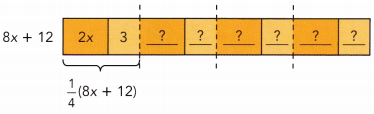From the bar model, $$\frac{1}{4}$$(8x +12) =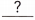Method 2
Use the distributive property.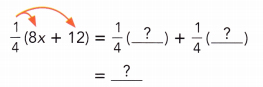$$\frac{1}{4}$$ (8x + 12) = 2x + 3.

Explanation:
Method 1:
$$\frac{1}{4}$$ (8x + 12)
= ($$\frac{1}{4}$$  × 8x) + ($$\frac{1}{4}$$  × 12)
= 2x + 3.
$$\frac{1}{4}$$ (8x + 12) = (2x + 3) + (2x + 3) +  (2x + 3) +  (2x + 3)

Method 2:
$$\frac{1}{4}$$ (8x + 12)
= ($$\frac{1}{4}$$  × 8x) + ($$\frac{1}{4}$$  × 12)
= 2x + 3.

Expand each expression.
Question 2.
$$\frac{1}{3}$$(9x + 6)
$$\frac{1}{3}$$(9x + 6) = 3x + 2.

Explanation:
$$\frac{1}{3}$$(9x + 6)
= ($$\frac{1}{3}$$ × 9x) + ($$\frac{1}{3}$$ × 6)
= 3x + 2

Question 3.
$$\frac{1}{5}$$(25x + 15)
$$\frac{1}{5}$$(25x + 15) = 5x + 3.

Explanation:
$$\frac{1}{5}$$(25x + 15)
= ($$\frac{1}{5}$$ × 25x) + ($$\frac{1}{5}$$ × 15)
= 5x + 3.

Copy and complete to expand each expression. Write + or – in each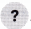.
Question 4.
0.3(2x + 5)
0.3(2x + 5) = 0.3() + 0.3()
=+0.3(2x + 5) = (0.3 × 2x) + (0.3 × 5) = 0.6x + 1.5.

Explanation:
0.3(2x + 5) = (0.3 × 2x) + (0.3 × 5)
= 0.6x + 1.5.

Question 5.
0.5(1.4y – 2.1)
0.5(1.4y – 2.1) = 0.5 ()0.5(-)
=(-)
=0.5(1.4y – 2.1) = (0.5 × 1.4y) + (0.5 × -2.1) = 0.7y – 1.05.

Explanation:
0.5(1.4y – 2.1) = (0.5 × 1.4y) + (0.5 × -2.1)
= 0.7y + (-1.05)
= 0.7y – 1.05.

Expand each expression.
Question 6.
0.4(3y + 2)
0.4(3y + 2) = 1.2y + 0.8.

Explanation:
0.4(3y + 2) = (0.4 × 3y) + (0.4 × 2)
= 1.2y + 0.8.

Question 7.
0.2(4x – 3.1)
0.2(4x – 3.1) = 0.8x – 6.2.

Explanation:
0.2(4x – 3.1) = (0.2 × 4x) + (0.2 × -3.1)
= 0.8x + (-6.2)
= 0.8x – 6.2.

Expand each expression.
Question 8.
-4(3d – 2)
-4(3d – 2) = -12d + 8.

Explanation:
-4(3d – 2) = (-4 × 3d) + (-4 × -2)
= -12d + 8.

Question 9.
-7(5k + e)
-7(5k + e) = -35k – 7e.

Explanation:
-7(5k + e) = (-7 × 5k) + (-7 × e)
= -35k + (-7e)
= -35k – 7e.

Question 10.
-4(0.6x – 4)
-4(0.6x – 4) = -2.4x + 16.

Explanation:
-4(0.6x – 4) = (-4 × 0.6x) + (-4 × -4)
= -2.4x + (16)
= -2.4x + 16.

Question 11.
–$$\frac{1}{4}$$(-3y + $$\frac{1}{2}$$)
–$$\frac{1}{4}$$(-3y + $$\frac{1}{2}$$) = $$\frac{3}{4}$$y –$$\frac{1}{8}$$

Explanation:
–$$\frac{1}{4}$$(-3y + $$\frac{1}{2}$$)
= (-$$\frac{1}{4}$$ × -3y) + (-$$\frac{1}{4}$$ × $$\frac{1}{2}$$))
= $$\frac{3}{4}$$y +(-$$\frac{1}{8}$$)
= $$\frac{3}{4}$$y –$$\frac{1}{8}$$

Copy and complete to expand and simplify the expression.
Question 12.
2(2a + 3b) + 5b .
2(2a + 3b) + 5b = 2(2 a) + 2 () +=++=2(2a + 3b) + 5b = 4a + 11b.

Explanation:
2(2a + 3b) + 5b = (2 × 2a) + (2 × 3b) + 5b.
= 4a + 6b + 5b
= 4a + 11b.

Expand and simplify each expression.
Question 13.
-3($$\frac{1}{2}$$k – 4) – 2k
-3($$\frac{1}{2}$$k – 4) – 2k = –$$\frac{5}{2}$$k + 12.

Explanation:
-3($$\frac{1}{2}$$k – 4) – 2k
= ( -3 × $$\frac{1}{2}$$k) + (-3 × -4) – 2k
= –$$\frac{3}{2}$$k + 12 – 2k
= (-3k – 2k) ÷ 2 + 12
= –$$\frac{5}{2}$$k + 12.

Question 14.
5(2h – 3) – (2k – 1)
5(2h – 3) – (2k – 1) = 10h – 2k – 14.

Explanation:
5(2h – 3) – (2k – 1) = (5 × 2h) + (5 × -3) -2k + 1.
= 10h – 15 – 2k + 1
= 10h – 2k – 14.

### Math in Focus Course 2A Practice 3.4 Answer Key

Expand each expression.
Question 1.
$$\frac{1}{4}$$ (4x + 8)
$$\frac{1}{4}$$ (4x + 8) = x + 2.

Explanation:
$$\frac{1}{4}$$ (4x + 8)
= ($$\frac{1}{4}$$ × 4x) + ($$\frac{1}{4}$$ × 8)
= x + 2.

Question 2.
$$\frac{1}{3}$$ (6b + 9)
$$\frac{1}{3}$$ (6b + 9) = 2b + 3.

Explanation:
$$\frac{1}{3}$$ (6b + 9)
= ($$\frac{1}{3}$$  × 6b) + ($$\frac{1}{3}$$ × 9)
= 2b + 3.

Question 3.
$$\frac{1}{2}$$ (p + 2)
$$\frac{1}{2}$$ (p + 2) = $$\frac{1}{2}$$p + $$\frac{1}{4}$$

Explanation:
$$\frac{1}{2}$$ (p + 2)
= ($$\frac{1}{2}$$ × p) + ($$\frac{1}{2}$$ × 2)
= $$\frac{1}{2}$$p + $$\frac{1}{4}$$

Question 4.
$$\frac{1}{5}$$ (4a + 3)
$$\frac{1}{5}$$ (4a + 3) = $$\frac{4}{5}$$a + $$\frac{3}{5}$$

Explanation:
$$\frac{1}{5}$$ (4a + 3)
= ($$\frac{1}{5}$$  × 4a) + ($$\frac{1}{5}$$ × 3)
= $$\frac{4}{5}$$a + $$\frac{3}{5}$$

Question 5.
$$\frac{1}{2}$$(4k – 6)
$$\frac{1}{2}$$(4k – 6) = 2k – 3.

Explanation:
$$\frac{1}{2}$$(4k – 6)
= ($$\frac{1}{2}$$ × 4k) + ( $$\frac{1}{2}$$ × -6)
= (2k) + (-3)
= 2k – 3.

Question 6.
$$\frac{1}{3}$$ (16a – 8)
$$\frac{1}{3}$$ (16a – 8) = $$\frac{16}{3}$$a – $$\frac{8}{3}$$.

Explanation:
$$\frac{1}{3}$$ (16a – 8)
= ($$\frac{1}{3}$$  × 16a) + ( $$\frac{1}{3}$$  × -8)
= ($$\frac{16}{3}$$a) + (- $$\frac{8}{3}$$)
= $$\frac{16}{3}$$a – $$\frac{8}{3}$$

Question 7.
$$\frac{1}{3}$$ (5b – 1)
$$\frac{1}{3}$$ (5b – 1) = $$\frac{5}{3}$$b – $$\frac{1}{3}$$

Explanation:
$$\frac{1}{3}$$ (5b – 1)
= ( $$\frac{1}{3}$$ × 5b) + ( $$\frac{1}{3}$$ × -1)
= ($$\frac{5}{3}$$b) + (- $$\frac{1}{3}$$)
= $$\frac{5}{3}$$b – $$\frac{1}{3}$$

Question 8.
$$\frac{2}{5}$$ (k – 10)
$$\frac{2}{5}$$ (k – 10) = $$\frac{2}{5}$$k – 4.

Explanation:
$$\frac{2}{5}$$ (k – 10)
= ($$\frac{2}{5}$$ × k) + ( $$\frac{2}{5}$$  × -10)
= ($$\frac{2}{5}$$k) + (-4)
= $$\frac{2}{5}$$k – 4.

Question 9.
3(4x + 0.2)
3(4x + 0.2) = 12x + 0.6.

Explanation:
3(4x + 0.2)
= (3 × 4x) + (3 × 0.2)
= 12x + 0.6.

Question 10.
4(0.1y + 5)
4(0.1y + 5) = 0.4y + 20.

Explanation:
4(0.1y + 5)
= (4 × 0.1y) + ( 4 × 5)
= 0.4y + 20.

Question 11.
0.2(3x + 4)
0.2(3x + 4) = 0.6x + 0.8.

Explanation:
0.2(3x + 4)
= (0.2 × 3x) + (0.2 × 4)
= 0.6x + 0.8.

Question 12.
0.6(3h + 5)
0.6(3h + 5) = 1.8h + 3.0.

Explanation:
0.6(3h + 5)
= (0.6 × 3h) + (0.6 × 5)
= 1.8h + 3.0.

Question 13.
0.2(m – 3)
0.2(m – 3) = 0.2m – 0.6.

Explanation:
0.2(m – 3) = (0.2 × m) + ( 0.2 × -3)
= (0.2m) + (-0.6)
= 0.2m – 0.6.

Question 14.
0.3(p – 3)
0.3(p – 3) = 0.3p – 0.9.

Explanation:
0.3(p – 3)
= (0.3 × p) + (0.3 × -3)
= (0.3p) + (-0.9)
= 0.3p – 0.9.

Question 15.
0.4(1.5d + 0.5)
0.4(1.5d + 0.5) = 0.6d + 0.20.

Explanation:
0.4(1.5d + 0.5) = (0.4 × 1.5d) + (0.4 × 0.5)
= (0.6d) + (0.20)
= 0.6d + 0.20.

Question 16.
1.2(0.3x – 1.4)
1.2(0.3x – 1.4) = 0.36x – 1.68.

Explanation:
1.2(0.3x – 1.4)
= (1.2 × 0.3x) + (1.2 × -1.4)
= (0.36x) + (-1.68)
= 0.36x – 1.68.

Expand each expression with a negative factor.
Question 17.
-2(x + 1)
-2(x + 1) = -2x – 2.

Explanation:
-2(x + 1) = (-2 × x) + (-2 × 1)
= (-2x) + (-2)
= -2x – 2.

Question 18.
-3(2x + 5)
-3(2x + 5) = -6x – 15.

Explanation:
-3(2x + 5)
= (-3 × 2x) + (-3 × 5)
= (-6x) + (-15)
= -6x – 15.

Question 19.
-3(4a + 9b)
-3(4a + 9b) = -12a – 27b.

Explanation:
-3(4a + 9b)
= (-3 × 4a) + (-3 × 9b)
= (-12a) + (-27b)
= -12a – 27b.

Question 20.
-7(2k – h)
-7(2k – h) = -14k + 7h.

Explanation:
-7(2k – h)
= (-7 × 2k) + (-7 × -h)
= (-14k) + (7h)
= -14k + 7h.

Question 21.
-4(p + $$\frac{1}{2}$$)
-4(p + $$\frac{1}{2}$$) = -4p – 2.

Explanation:
-4(p + $$\frac{1}{2}$$) = (-4 × p) + (-4 × $$\frac{1}{2}$$)
= (-4p) + × (-2)
= -4p – 2.

Question 22.
–$$\frac{1}{2}$$ (6x – $$\frac{1}{3}$$)
–$$\frac{1}{2}$$ (6x – $$\frac{1}{3}$$) = -3x + $$\frac{1}{6}$$.

Explanation:
–$$\frac{1}{2}$$ (6x – $$\frac{1}{3}$$)
= (-$$\frac{1}{2}$$  × 6x) + (-$$\frac{1}{2}$$ × – $$\frac{1}{3}$$)
= (-3x) + ($$\frac{1}{6}$$)
= -3x + $$\frac{1}{6}$$.

Question 23.
-2(5k + 1.7)
-2(5k + 1.7) = -10k – 3.4.

Explanation:
-2(5k + 1.7) = (-2 × 5k) + (-2 × 1.7)
= (-10k) + (-3.4)
= -10k – 3.4.

Question 24.
-3(0.2m + 5)
-3(0.2m + 5) = -0.6m – 15.

Explanation:
-3(0.2m + 5)
= (-3 × 0.2m) + (-3 × 5)
= (-0.6m) + (-15)
= -0.6m – 15.

Question 25.
-5(q – 0.3)
-5(q – 0.3) = -5q + 1.5.

Explanation:
-5(q – 0.3)
= (-5 × q) + (-5 × -0.3)
= (-5q) + (1.5)
= -5q + 1.5.

Question 26.
-0.6(0.4y – 1)
-0.6(0.4y – 1) = -0.24y + 0.6.

Explanation:
-0.6(0.4y – 1)
= (-0.6 × 0.4y) + (-0.6 × -1)
= (-0.24y) + (0.6)
= -0.24y + 0.6.

Expand and simplify each expression.
Question 27.
3(2y + 1) + 4
3(2y + 1) + 4 = 6y + 7.

Explanation:
3(2y + 1) + 4
= (3 × 2y) + (3 × 1) + 4
= 6y + 3 + 4
= 6y + 7.

Question 28.
3(2a + 5) – 8
3(2a + 5) – 8 = 6a + 7.

Explanation:
3(2a + 5) – 8
= (3 × 2a) + (3 × 5) – 8
= 6a + 15 – 8
= 6a + 7.

Question 29.
2(x + 2) + 3x
2(x + 2) + 3x = 5x + 4.

Explanation:
2(x + 2) + 3x
= (2 × x) + (2 × 2) + 3x
= 2x + 4 + 3x
= 5x + 4.

Question 30.
6(b + 3) – 2b
6(b + 3) – 2b = 4b + 18.

Explanation:
6(b + 3) – 2b
= (6 × b) + (6 × 3) – 2b.
= 6b + 18 – 2b
= 4b + 18.

Question 31.
5($$\frac{1}{6}$$a + 1) + 3
5($$\frac{1}{6}$$a + 1) + 3 = $$\frac{5}{6}$$a  + 8.

Explanation:
5($$\frac{1}{6}$$a + 1) + 3
= (5 × $$\frac{1}{6}$$a) + (5 × 1) + 3
= ($$\frac{5}{6}$$a) + 5 + 3.
= $$\frac{5}{6}$$a + 5 + 3
= $$\frac{5}{6}$$a  + 8.

Question 32.
4($$\frac{1}{8}$$a – 3) – $$\frac{1}{2}$$a
4($$\frac{1}{8}$$a – 3) – $$\frac{1}{2}$$a = -12.

Explanation:
4($$\frac{1}{8}$$a – 3) – $$\frac{1}{2}$$a
= (4 × $$\frac{1}{8}$$a) + (4 × -3) – $$\frac{1}{2}$$a
= $$\frac{1}{2}$$a – 12 – $$\frac{1}{2}$$a
= -12.

Expand and simplify each expression.
Question 33.
0.2(x + 1) + 0.7x.
0.2(x + 1) + 0.7x. = 0.9x + 0.2.

Explanation:
0.2(x + 1) + 0.7x
= (0.2 × x) + (0.2 × 1) + 0.7x
= 0.2x + 0.2 + 0.7x
= 0.9x + 0.2.

Question 34.
0.5(y + 2) – 0.3y
0.5(y + 2) – 0.3y = 0.2y + 1.0.

Explanation:
0.5(y + 2) – 0.3y
= (0.5 × y) + ( 0.5 × 2) – 0.3y.
= 0.5y + 1.0 – 0.3y
= 0.2y + 1.0.

Question 35.
-2(4 m + 1) – m
-2(4 m + 1) – m = -9m – 2.

Explanation:
-2(4 m + 1) – m
= (-2 × 4m) + (-2 × 1) -m
= -8m – 2 – m
= -9m – 2.

Question 36.
10 – 3(2n – 1)
10 – 3(2n – 1) = 13 – 6n.

Explanation:
10 – 3(2n – 1)
= 10 + (-3 × 2n) + (-3 × -1)
= 10 – 6n + 3
= 13 – 6n.

Question 37.
-0.8(r + 3) + 2.2r
-0.8(r + 3) + 2.2r = 1.4r – 2.4.

Explanation:
-0.8(r + 3) + 2.2r
= (-0.8 × r) + (-0.8 × 3) + 2.2r
= -0.8r – 2.4 + 2.2r
= 1.4r – 2.4.

Question 38.
-(1.2x + 7) + 1.5x
-(1.2x + 7) + 1.5x = -7 + 0.3x.

Explanation:
-(1.2x + 7) + 1.5x
= (-1 × 1.2x) + (-1 × 7) + 1.5x
= -1.2x – 7 + 1.5x
= -7 + 0.3x.

Expand and simplify each expression with two variables.
Question 39.
4x + 6(3y + x)
4x + 6(3y + x) = 10x + 18y.

Explanation:
4x + 6(3y + x)
= 4x + (6 × 3y) + (6 × x)
= 4x + 18y + 6x
= 10x + 18y.

Question 40.
7a + 5(3a – b)
7a + 5(3a – b) = 22a – 5b.

Explanation:
7a + 5(3a – b)
= 7a + (5 × 3a) + (5 × -b)
= 7a + 15a – 5b
= 22a – 5b.

Question 41.
8g + 5(v – g)
8g + 5(v – g) = 3g + 5v.

Explanation:
8g + 5(v – g)
= 8g + (5 × v) + (5 × -g)
= 8g + 5v – 5g
= 3g + 5v.

Question 42.
4q + 6(p – 2q)
4q + 6(p – 2q) = 6p – 8q.

Explanation:
4q + 6(p – 2q) = 4q + (6 × p) + (6 × -2q)
= 4q + 6p – 12q
= 6p – 8q.

Question 43.
2(a + 2b) + (a + 3b)
2(a + 2b) + (a + 3b) = 3a + 7b.

Explanation:
2(a + 2b) + (a + 3b)
= (2 × a) + (2 × 2b) + a + 3b
= 2a + 4b + a + 3b
= 3a + 7b.

Question 44.
3(m – 2n) + 6(n – 2m)
3(m – 2n) + 6(n – 2m) = -9m.

Explanation:
3(m – 2n) + 6(n – 2m) = (3 × m) + (3 × -2n) + (6 ×n) + (6 × -2m)
= 3m – 6n + 6n – 12m
= -9m.

Question 45.
4(d + e) – 3(d – 2e)
4(d + e) – 3(d – 2e) = d + 10e.

Explanation:
4(d + e) – 3(d – 2e)
= (4 × d) + (4 × e) + (-3 × d) + (-3 × -2e)
= 4d + 4e – 3d + 6e
= d + 10e.

Question 46.
3(3q – p) – (q – 6p)
3(3q – p) – (q – 6p) = 8q + 3p.

Explanation:
3(3q – p) – (q – 6p)
= (3 × 3q) + (3 × -p) + (-1 × q) + (-1 × -6p)
= 9q – 3p – q + 6p
= 8q + 3p.

Question 47.
-4(x + 3y) + 3(2x – 5y)
-4(x + 3y) + 3(2x – 5y) = 2x – 27y.

Explanation:
-4(x + 3y) + 3(2x – 5y)
= (-4 × x) + (-4 × 3y) + (3 × 2x) + (3 × -5y)
= -4x – 12y + 6x – 15y
= 2x – 27y.

Question 48.
-7(y + 2t) – 3(y – t)
-7(y + 2t) – 3(y – t) = -10y – 11t.

Explanation:
-7(y + 2t) – 3(y – t)
= (-7 × y) + (-7 × 2t) + (-3 5 y) + (-3 × -t)
= -7y – 14t -3y + 3t
= -10y – 11t.

Write an expression for the missing dimension of each shaded figure and a multiplication expression for its area. Then expand and simplify the multiplication expression.
Question 49.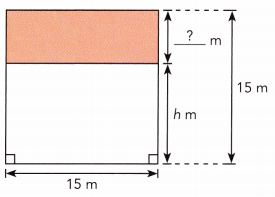Area of the ABCD square figure = 225 square m.

Explanation: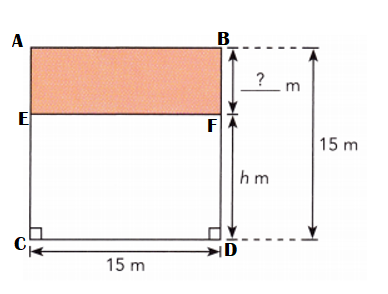Length of BF shaded figure = BD – BF
= 15 m – h m
= (15 – h) m.
Area of the ABCD square figure = CD × BD
= 15 m × 15 m
= 225 square m.

Question 50.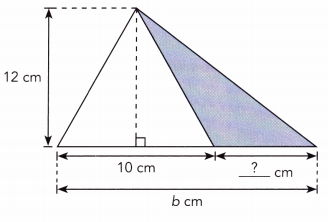Area of triangle ADE = 6b square cm.

Explanation: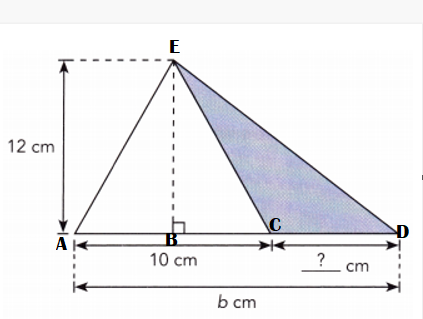Length of the CD shaded figure :
= b cm = 10 cm + ?? cm
= b cm – 10cm = ?? cm.
Area of triangle ADE = $$\frac{1}{2}$$ × base × height
= $$\frac{1}{2}$$ × b cm × 12 cm
= 6cm × b cm
= 6b square cm.

Write an expression for the area of the figure. Expand and simplify.
Question 51.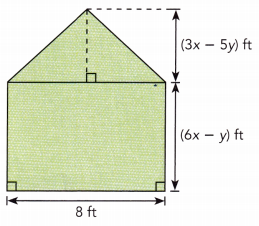Total area of ABCDE figure = (60x – 28y) ft.

Explanation: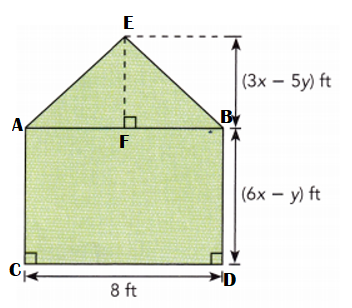Area of the triangle ABE = $$\frac{1}{2}$$ × base × height
= $$\frac{1}{2}$$  × 8 ft × (3x – 5y) ft
= 4 ft × (3x – 5y) ft
= (4 × 3x) + (4 × -5y) ft
= (12x – 20y) ft
Area of rectangle ABCD = Length × Width
= 8 ft × ((6x – y) ft
= [(8 × 6x) + (8 × -y)] ft
= (48x – 8y) ft
Total area of ABCDE = Area of the triangle ABE + Area of rectangle ABCD
= (12x – 20y) ft + (48x – 8y) ft
= (12x – 20y + 48x – 8y) ft
= (60x – 28y) ft.